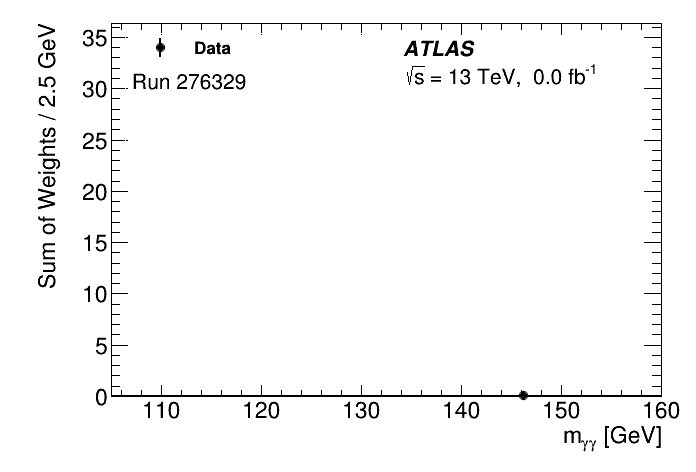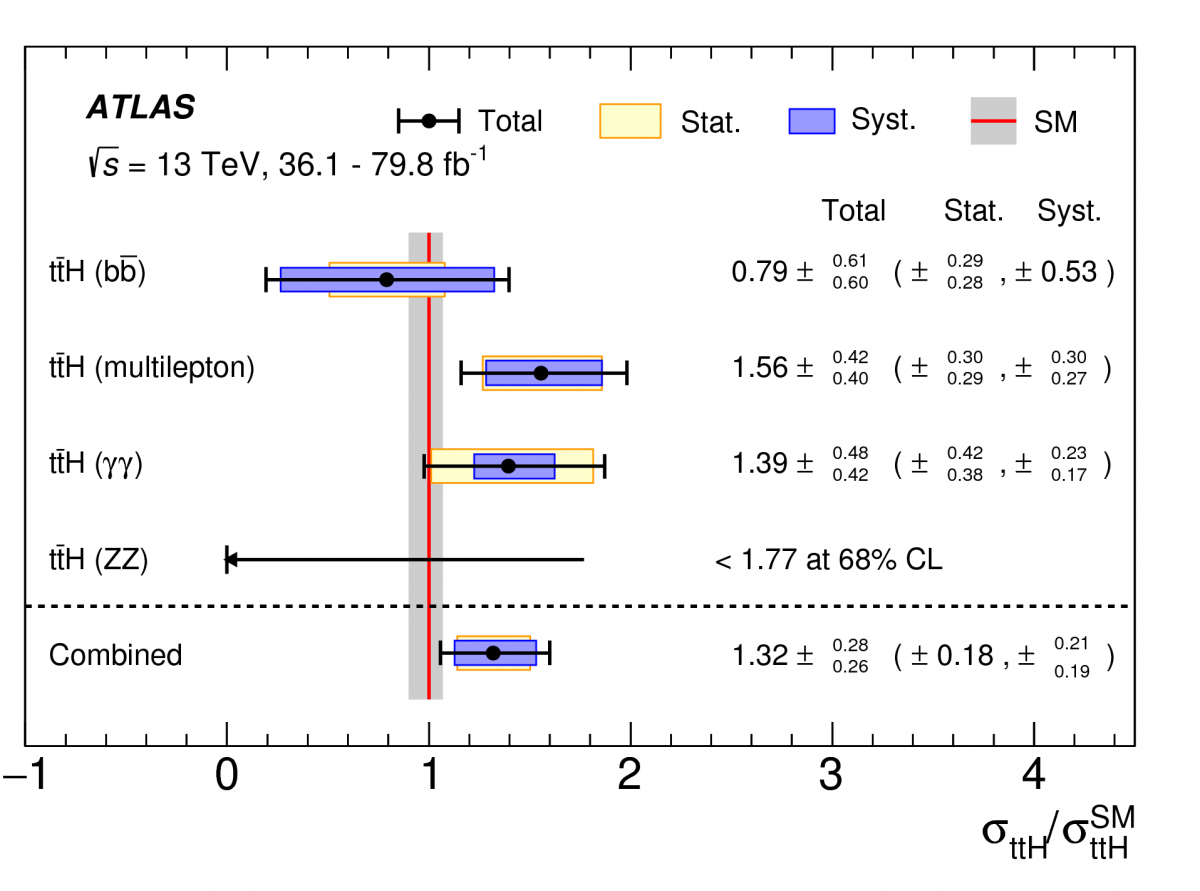Tags:

# New ATLAS result establishes production of Higgs boson in association with top quarks

This rare process is one of the most sensitive tests of the Higgs mechanism

4 June 2018 | By

According to the Standard Model, quarks, charged leptons, and W and Z bosons obtain their mass through interactions with the Higgs field, a quantum fluctuation of which gives rise to the Higgs boson. To test this theory, ATLAS takes high-precision measurements of the interactions between the Higgs boson and these particles. While the ATLAS and CMS experiments at CERN’s Large Hadron Collider (LHC) had observed and measured the Higgs boson decaying to pairs of W or Z bosons, photons or tau leptons, the Higgs coupling to quarks had not – despite evidence – been observed.

In results presented today at the LHCP2018 conference, the ATLAS Collaboration has observed the production of the Higgs boson together with a top-quark pair (known as “ttH” production). Only about 1% of all Higgs bosons are produced through this rare process. This result establishes a direct measurement of the interaction between the top quark and the Higgs boson (known as the “top quark Yukawa coupling”). As the top quark is the heaviest particle in the Standard Model, this measurement is one of the most sensitive tests of the Higgs mechanism.

### This new ATLAS result establishes a direct measurement of the interaction between the top quark and the Higgs boson.

Previous ATLAS measurements using 2015 and 2016 data provided the first evidence for ttH production from a combination of channels where the Higgs boson decayed to two W or Z bosons (WW* or ZZ*), to a pair of tau leptons, to a pair of b-quarks, or to a pair of photons (“diphoton”). Those results have now been updated with the measurements of the diphoton and ZZ* decay modes that use the larger 2015-2017 dataset, and where improved reconstruction algorithms and new analysis techniques have increased the sensitivity of the measurements. The CMS Collaboration recently reported the observation of ttH production by combining 2015 and 2016 data with data taken at lower collision energies in earlier LHC runs.

### Evidence for ttH production in the diphoton channel in the 2015-2017 dataset

The probability of a Higgs boson decaying to a diphoton pair is only about 0.2%, making the predicted rate for ttH production in this channel quite small. However, because the energy and direction of photons can be well measured with the ATLAS detector, the reconstructed mass peak obtained with this decay mode is narrow. It is therefore possible to observe a signal even when the number of events is low. Furthermore, regions with lower and higher reconstructed mass (called the “sidebands”) can be used to estimate the background under the signal peak using the data themselves, rendering this channel particularly robust.

To optimize the measurement ATLAS employs machine learning techniques. Events consistent with the ttH kinematics are selected using “boosted decision tree” (BDT) algorithms that allow physicists to separate the events into multiple categories with different signal-to-background abundance ratios. Depending on the top-quark decay channel considered, the inputs given to the BDT are the momenta of the “jets” (collimated groups of particles that are produced by a quark or gluon), leptons and photons observed in each event. As the decay of a top quark always produces a b-quark, identifying jets that arise from b-quarks is essential for reducing backgrounds. To achieve this, ATLAS developed a b-identification algorithm (also based on machine learning); the b-identification decision for each jet is included in the BDT inputs.Figure 1: Time-lapse animation showing the increasing ttH signal in the diphoton mass spectrum as more data are included in the measurement. (Image: ATLAS Collaboration/CERN)

Each category is analysed separately by studying the distribution of the invariant mass of the diphoton candidates in selected events. This distribution is fit to a combination of signal (Higgs boson decay to diphoton in events containing a top-quark pair) and background (cases where the diphoton candidate does not arise from a Higgs boson or where the event does not contain a true top-quark pair). The numbers of fitted signal events in the different categories are then statistically combined, taking into account correlated experimental and theoretical systematic uncertainties.

The result of the above procedure, using 80 fb-1 of data recorded in 2015, 2016 and the recent 2017 run of the LHC, is summarised in Figure 1, which shows the diphoton invariant mass distribution, summed over categories weighted by their signal purity. The significance of the observed signal is 4.1 standard deviations; the expected significance for Standard Model production is 3.7 standard deviations.

### Search continues for ttH production in the ZZ* channel in the 2015-2017 dataset

The decay of a Higgs boson to ZZ* with the subsequent decay of the ZZ* to four leptons is another channel where the Higgs mass peak is narrow. Due to the very clean detector signature of the four-lepton decay mode, this channel is essentially free of backgrounds apart from small contributions from Higgs bosons produced through other production modes than ttH. However, this decay mode is even rarer than that of diphotons, with less than one event expected from ttH production in the 80 fb-1 of the full 2015-2017 dataset. A dedicated search for this decay was performed, but no candidate events were found in the 2015-2017 ATLAS data.Figure 2: Combined ttH production cross section, as well as cross sections measured in the individual analyses, divided by the SM cross section prediction. ML indicates the analysis of the two and three lepton final states (multilepton). The black lines show the total uncertainties, while the bands indicate the statistical and systematic uncertainties. The red line indicates the SM cross section prediction, and the grey band represents the theoretical uncertainties on the prediction. For the γγ and ZZ* channels to full dataset at 13 GeV (collected between 2015 and 2017) have been used, whereas the results of the other channels are based on the 2015 and 2016 data. (Image: ATLAS Collaboration/CERN)

### Combination with earlier ATLAS results

The measurements described above have been combined with the previously reported searches for ttH that used 2015 and 2016 data. Decays of the Higgs boson to a b-quark pair and to a pair of W bosons or tau leptons had observed (expected) significances of 1.4 (1.6) and 4.2 (2.8) standard deviations, respectively.

After the combination, the observed (expected) significance of the signal over the background is 5.8 (4.9) standard deviations. The ratio of the combined ttH cross section measurement and the cross section measurements separated by Higgs boson decay modes are presented in Figure 2. The measured ratio of 1.32 ± 0.27 is slightly larger than, but consistent with the Standard Model expectation.

Further searches for the ttH process were performed using 7 and 8 TeV data collected during Run 1. When combined with the 2015-2017 results, the observed (expected) significance is 6.3 (5.1) standard deviations.

### Summary

ATLAS has observed the production of the Higgs boson in association with a top-quark pair with a significance of 6.3 standard deviations over the background-only hypothesis. The measured ttH production cross section is consistent with the Standard Model prediction. This measurement provides direct evidence for the coupling of the Higgs boson to the top quark and supports the Standard Model mechanism whereby the top quark obtains its mass through interaction with the Higgs field.